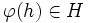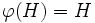Difference between revisions of "Injective endomorphism-invariant subgroup"

BEWARE! This term is nonstandard and is being used locally within the wiki. [SHOW MORE]
This article defines a subgroup property: a property that can be evaluated to true/false given a group and a subgroup thereof, invariant under subgroup equivalence. View a complete list of subgroup properties[SHOW MORE]
If the ambient group is a finite group, this property is equivalent to the property: characteristic subgroup
View other properties finitarily equivalent to characteristic subgroup | View other variations of characteristic subgroup | Read a survey article on varying characteristic subgroup

Definition

QUICK PHRASES: invariant under all injective endomorphisms, injective endomorphism-invariant

Equivalent definitions in tabular format

Below are many equivalent definitions of injective endomorphism-invariant subgroup.

No. Shorthand A subgroup of a group is characteristic in it if... A subgroup$H$ of a group$G$ is called a characteristic subgroup of$G$ if ...
1 injective endomorphism-invariant every injective endomorphism of the whole group takes the subgroup to within itself for every injective endomorphism$\varphi$ of$G$,$\varphi(H) \subseteq H$. More explicitly, for any$h \in H$ and$\varphi \in \operatorname{End}(G)$ that is injective,$\varphi(h) \in H$
2 injective endomorphisms restrict to endomorphisms every injective endomorphism of the group restricts to an endomorphism of the subgroup. for every injective endomorphism$\varphi$ of$G$,$\varphi(H) \subseteq H$ and$\varphi$ restricts to an endomorphism of$H$
3 injective endomorphisms restrict to injective endomorphisms every injective endomorphism of the group restricts to an injective endomorphism of the subgroup for every injective endomorphism$\varphi$ of$G$,$\varphi(H) = H$ and$\varphi$ restricts to an injective endomorphism of$H$
This definition is presented using a tabular format. |View all pages with definitions in tabular format

Formalisms

Function restriction expression

This subgroup property is a function restriction-expressible subgroup property: it can be expressed by means of the function restriction formalism, viz there is a function restriction expression for it.
Find other function restriction-expressible subgroup properties | View the function restriction formalism chart for a graphic placement of this property
Function restriction expression$H$ is an injective endomorphism-invariant subgroup of$G$ if ... This means that injective endomorphism-invariance is ... Additional comments
injective endomorphism$\to$ function every injective endomorphism of$G$ sends every element of$H$ to within$H$ the invariance property for injective endomorphisms
injective endomorphism$\to$ endomorphism every injective endomorphism of$G$ restricts to an endomorphism of$H$ the endo-invariance property for injective endomorphisms; i.e., it is the invariance property for injective endomorphism, which is a property stronger than the property of being an endomorphism
injective endomorphism$\to$ injective endomorphism every injective endomorphism of$G$ restricts to a injective endomorphism of$H$ the balanced subgroup property for injective endomorphisms Hence, it is a t.i. subgroup property, both transitive and identity-true

Relation with other properties

Stronger properties

Property Meaning Proof of implication Proof of strictness (reverse implication failure) Intermediate notions
fully invariant subgroup |FULL LIST, MORE INFO
isomorph-free subgroup Intermediately injective endomorphism-invariant subgroup, Isomorph-containing subgroup|FULL LIST, MORE INFO
isomorph-containing subgroup Intermediately injective endomorphism-invariant subgroup|FULL LIST, MORE INFO
intermediately injective endomorphism-invariant subgroup |FULL LIST, MORE INFO

Weaker properties

Property Meaning Proof of implication Proof of strictness (reverse implication failure) Intermediate notions
characteristic subgroup invariant under all automorphisms injective endomorphism-invariant implies characteristic characteristic not implies injective endomorphism-invariant |FULL LIST, MORE INFO
normal subgroup invariant under all inner automorphisms (via characteristic) (via characteristic) Characteristic subgroup|FULL LIST, MORE INFO

Metaproperties

Transitivity

This subgroup property is transitive: a subgroup with this property in a subgroup with this property, also has this property in the whole group.
ABOUT THIS PROPERTY: View variations of this property that are transitive | View variations of this property that are not transitive
ABOUT TRANSITIVITY: View a complete list of transitive subgroup properties|View a complete list of facts related to transitivity of subgroup properties |Read a survey article on proving transitivity

The property of being injective endomorphism-invariant is transitive on account of its being a balanced subgroup property (function restriction formalism).

For full proof, refer: Injective endomorphism-invariance is transitive

Further information: Balanced implies transitive, full invariance is transitive, characteristicity is transitive

Trimness

This subgroup property is trim -- it is both trivially true (true for the trivial subgroup) and identity-true (true for a group as a subgroup of itself).
View other trim subgroup properties | View other trivially true subgroup properties | View other identity-true subgroup properties

The trivial subgroup is injective endomorphism-invariant because every endomorphism (injective or not) must take it to itself.

The whole group is also clearly injective endomorphism-invariant.

Intersection-closedness

YES: This subgroup property is intersection-closed: an arbitrary (nonempty) intersection of subgroups with this property, also has this property.
ABOUT THIS PROPERTY: View variations of this property that are intersection-closed | View variations of this property that are not intersection-closed
ABOUT INTERSECTION-CLOSEDNESS: View all intersection-closed subgroup properties (or, strongly intersection-closed properties) | View all subgroup properties that are not intersection-closed | Read a survey article on proving intersection-closedness | Read a survey article on disproving intersection-closedness

An arbitrary intersection of injective endomorphism-invariant subgroups is injective endomorphism-invariant. This follows on account of injective endomorphism-invariance being an invariance property.

For full proof, refer: Injective endomorphism-invariance is strongly intersection-closed

Join-closedness

YES: This subgroup property is join-closed: an arbitrary (nonempty) join of subgroups with this property, also has this property.
ABOUT THIS PROPERTY: View variations of this property that are join-closed | View variations of this property that are not join-closed
ABOUT JOIN-CLOSEDNESS: View all join-closed subgroup properties (or, strongly join-closed properties) | View all subgroup properties that are not join-closed | Read a survey article on proving join-closedness | Read a survey article on disproving join-closedness

An arbitrary join of injective endomorphism-invariant subgroups is injective endomorphism-invariant. This follows on account of injective endomorphism-invariance being an endo-invariance property.

For full proof, refer: Injective endomorphism-invariance is strongly join-closed